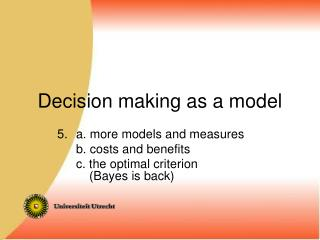DownloadDownload PresentationDecision making as a model

# Decision making as a model

Télécharger la présentation## Decision making as a model

- - - - - - - - - - - - - - - - - - - - - - - - - - - E N D - - - - - - - - - - - - - - - - - - - - - - - - - - -
##### Presentation Transcript

1. Decision making as a model a. more models and measures b. costs and benefits c. the optimal criterion (Bayes is back)

2. Unequal variances:

3. Different d'-s (model equal variances) A B Same sensitivity? (model uneqal variances)

4. zFA μs zH = ---- + --- σsσs μs – λμs – λ PH = ΦzH = σsσs Unequal variance model σn=1, σs PH PFA zH 0 λμs μs/σs PFA = Φ(-λ), zFA = -λ θ -μs zFA tg(θ) = 1/σs

5. μs √1 + σs2 Δm does not distinguish between large and small σs Measures: zH distance to origin analogous to d' : ZH= -ZFA de = Oe√2 da = Oa√2 e a zFA O Δm (Pythagoras and similar triangles)

6. To get Az , the surface under the ROC-curve according to the Gaussian model with unequal variances: Produce a formula for the proportion correct 2AFC-experiment under that model: PCZ According to the area theorem PC equals A so PCZ equals Az

7. PH Area under Gaussian ROC-curve: Az PFA Gaussian 2AFC: n s PC = p(xs>xn) = p(xs-xn>0)

8. PCZ = p(xs>xn) = p(xs- xn>0) μs = Φ √1 + σs2 -μs =1 - Φ √1 + σs2 The variance of the difference of two independent random variables is the sum of both variances -μs = Az according to area theorem!

9. μs = Φ √1 + σs2 μs/σs = Φ √1/σs2 + 1 PH Area under Gaussian ROC-curve: Az (already shown) PFA tg Az = Φ(da /√2) Equal variances : Az= Ad' = Φ(d′ /√2)

10. μs – λμs – λ PH = ΦzH = σsσs β with unequal variances: h / f = fs(λ) / fn(λ) f So: βunequal= βequal / σs h λ 1 -(λ – μs )2 /2σ2 1 -zH2/2 fs(λ) = ------------ e = ------------ e σs√(2π) σs√ (2π) 1 (zFA2 – zH2)/2 Divide: ------------------------- = --- e σs 1 -λ2/2 1 -zFA2/2 fn(λ) = --------- e = --------- e √(2π) √ (2π) zH (from slide 5) μs/σs PFA = Φ(-λ), zFA = -λ θ -μs zFA tg(θ) = 1/σs

11. Survey of signal detection measures General. Rough Gaussian many pts (one pt)σn ≠ σsσn = σs Sensitivity Criterium/bias With these measures the sensitivity and the criteria of humans, machines and systems can be expressed independently

12. What are the costs of missing a weapon/explosive at an airport? What are the costs of a false alarm? What are the costs and benefits of baggage screening?

13. Costs and benefits: Pay-off matrix “no” “yes” NB. C is a positive number: “a false alarm will cost you € 5” S(+N) N NB.: PH∙p(s)! EV = p(Hit)•Vhit - p(Miss)•CMiss+ p(CR)•VCR - p(FA)•CFA = p(s)•{PH• VHit– (1-PH)•CMiss} +p(n)•{(1-PFA)•VCR-PFA•CFA} - Cscr Compare with doing nothing: EV = p(n)•VCR– p(s)CMiss NB.: no free lunch, no free screening!

14. An optimal decison in uncertainty: xc x Set criterion at the value of x (xc) at which expected value/utility of “Yes” equals expected value/utility of “No” EV(Yes|xc) = EV(No|xc)

15. EV(Yes|xc) = EV(No|xc) “cost”: CFA positive! VHit• p(Hit) – CFA• p(FA) = VCR•p(CR) - CMiss•p(Miss) VHit• p(signal|xc) – CFA• p(noise|xc) = VCR•p(noise|xc) - CMiss•p(signal|xc) VHit•p(signal|xc) + CMissp(signal|xc) = VCR•p(noise|xc) + CFA•p(noise|xc) p(signal|xc)(VHit + CMiss) = p(noise|xc)(VCR + CFA) p(signal|xc) VCR + CFA ---------------- = --------------- p(noise|xc) VHit + CMiss But do we know that one?

16. p(signal|xc) VCR + CFA ---------------- = --------------- p(noise|xc) VHit + CMiss We want this one p(x|noise) We know (in principle): p(x|signal) required: a way to get from p(A|B) to p(B|A) Bayes’ Rule!

17. p(xc|signal) p(signal) • p(xc|noise) p(noise) p(A|B) p(B|A) p(A) --------- = ---------- • ------- p(A|¬B) p(B|¬A) p(¬A) (odds form) Applied to signal detection: p(signal|xc) --------------- p(noise|xc) =

18. p(signal|xc) VCR + CFA ---------------- = --------------- p(noise|xc) VHit + CMiss Bayes p(xc|signal) p(signal) VCR + CFA-------------- • --------- --- = --------------- p(xc|noise) p(noise) VHit + CMiss p(xc|signal) p(noise) VCR+CFA ---------------- = ------------- •-------------p(xc|noise) p(signal) VHit+CMiss LRc prior odds payoff matrix S, β

19. p(xc|signal) p(noise) VCR+CFA ---------------- = ------------- •-------------p(xc|noise) p(signal) VHit+CMiss So: an ideal observator, knowing prior odds and pay-off matrix, can compute an optimal criterion. People are not that good at arithmetic, but adapt reasonably well to pay-off matrix and prior odds

20. Nog meer weten over signaaldetectie? Wickens, T. D.(2002). Elementary signal detection theory. Oxford University Press. Macmillan, N. A. & Creelman C. D.(2005). Detection Theory: A user’s guide, 2nd ed. New York: Lawrence Erlbaum Associates